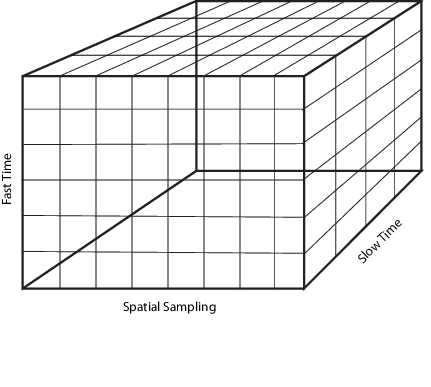The radar data cube is a convenient way to conceptually represent space-time processing. To construct the radar data cube, assume that preprocessing converts the RF signals received from multiple pulses across multiple array elements to complex-valued baseband samples. Arrange the complex-valued baseband samples in a three-dimensional array of size K-by-N-by-L.

• K defines the length of the first (fast-time) dimension.

• N defines the length of the second (spatial) dimension.

• L defines the length of the third (slow-time) dimension.

Many radar signal processing operations in Phased Array System Toolbox™ software correspond to processing lower-dimensional subsets of the radar data cube. The subset could be a one-dimensional subvector or a two-dimensional submatrix.

The following figure shows the organization of the radar data cube in this software. Subsequent sections explain each of the dimensions and which aspect of space-time processing they represent.### Fast Time Samples

Consider an K-by-1 subvector of the radar data cube along the fast-time axis in the preceding diagram. Each column vector represents a set of complex-valued baseband samples from a single pulse at one array element sampled at the rate${F}_{s}$. This sampling rate is the highest sampling rate of the system and leads to the designation fast time. Choose the sampling rate, ${F}_{s}$, large enough to avoid aliasing. The corresponding sampling interval is ${T}_{s}=1/{F}_{s}$. The fast time dimension is also referred to as the range dimension. Fast time sample intervals, when converted to distance using the signal propagation speed, are often referred to as range bins, or range gates.

Pulse compression is an example of a signal processing operation performed on the fast time samples. Another example of signal processing is dechirping. In these types of operations, the number of samples in the first dimension of the output can differ from the input.

### Slow Time Samples

Consider each K-by-L submatrix of the radar data cube. The submatrix contains K row vectors with dimension 1-by-L. Each of these row vectors contains complex-valued baseband samples from L different pulses from the same range bin. There is a K-by-L matrix for each of the N array elements. The sampling interval between the L samples is the pulse repetition interval (PRI). Typical PRIs are much longer than the fast-time sampling interval. Because of the long sampling intervals, samples taken across multiple pulses are referred to as slow time.

Processing data in the slow-time dimension allows you to estimate the Doppler spectrum at a given range bin. In this type of operation, the number of samples in the third dimension of the data cube can change. The number of Doppler bins is not necessarily equal to the number of pulses.

The Nyquist criterion applies equally to the slow-time dimension. The reciprocal of the PRI is the pulse repetition frequency (PRF). The PRF gives the width of the unambiguous Doppler spectrum.

### Spatial Sampling

Phased arrays consist of multiple array elements. Consider each K-by-N submatrix of the radar data cube. Each column vector consists of K fast-time samples for a single pulse received at a single array element. The N column vectors represent the same pulse sampled across N array elements. The sampled data in the N column vectors is a spatial sampling of the incident waveform. Analysis of the data across array elements lets you determine the spatial frequency content of each received pulse. The Nyquist criterion for spatial sampling requires that array elements not be separated by more than one-half the wavelength of the carrier frequency.

In spatial frequency operations, the number of samples in the second dimension of the data cube can change. The number of spatial frequency bins is not necessarily equal to the number of sensor elements.

Beamforming is a spatial filtering operation that combines data across the array elements to selectively enhance and suppress wavefields incident on the array from particular directions.

### Space-Time Processing

Space-time adaptive processing operates on the two-dimensional angle-Doppler data for each range bin. Consider the K-by-N-by-L radar data cube. Each of the K samples is data from the same range. This range is sampled across N array elements, and L PRIs. Collapsing the three-dimensional matrix at each range bin into N-by-L submatrices allows the simultaneous two-dimensional analysis of angle of arrival and Doppler frequency.

### Organizing Data in the Radar Data Cube

If you have K complex-valued baseband data samples collected from L pulses received at N sensors, you can organize your data in a format compatible with the Phased Array System Toolbox conventions using `permute`. After processing your data, you can convert back to the original data cube format with `ipermute`.

#### Reordering the Data Cube

Start with a data set consisting of 200 samples per pulse for ten pulses collected at 6 sensor elements. Your data is organized as a 6-by-10-by-200 MATLAB® array. Reorganize the data into a Phased Array System Toolbox™ compatible data cube.

Simulate this data structure using complex-valued white Gaussian noise samples.

`origdata = randn(6,10,200)+1j*randn(6,10,200);`

The first dimension of `origdata` is the number of sensors (spatial sampling), the second dimension is the number of pulses (slow-time), and the third dimension contains the fast-time samples. Phased Array System Toolbox™ expects the first dimension to contain the fast-time samples, the second dimension to represent individual sensors in the array, and the third dimension to contain the slow-time samples.

To reorganize `origdata` into a format compatible with the toolbox conventions, enter:

`newdata = permute(origdata,[3 1 2]);`

The `permute` function moves the third dimension of `origdata` into the first dimension of `newdata`. The first dimension of `origdata` becomes the second dimension of `newdata` and the second dimension of `origdata` becomes the third dimension of `newdata`. This results in `newdata` being organized as fast-time samples-by-sensors-by-slow-time samples. You can now process `newdata` with Phased Array System Toolbox functions.

After you process your data, you can use `ipermute` to return your data to the original structure.

`data = ipermute(newdata,[3 1 2]);`

In this case, `data` is the same as `origdata`.